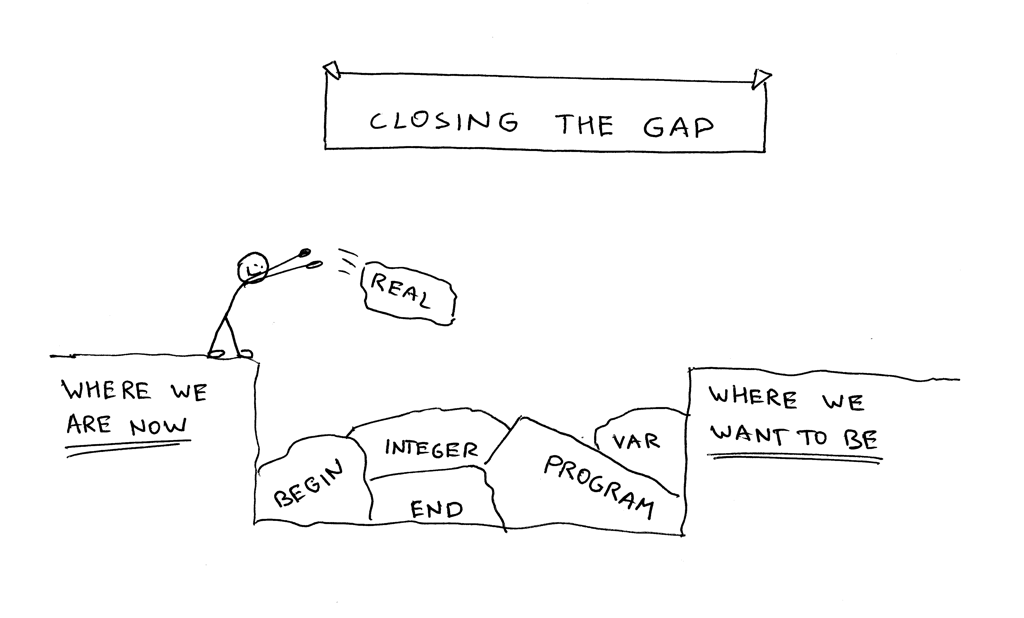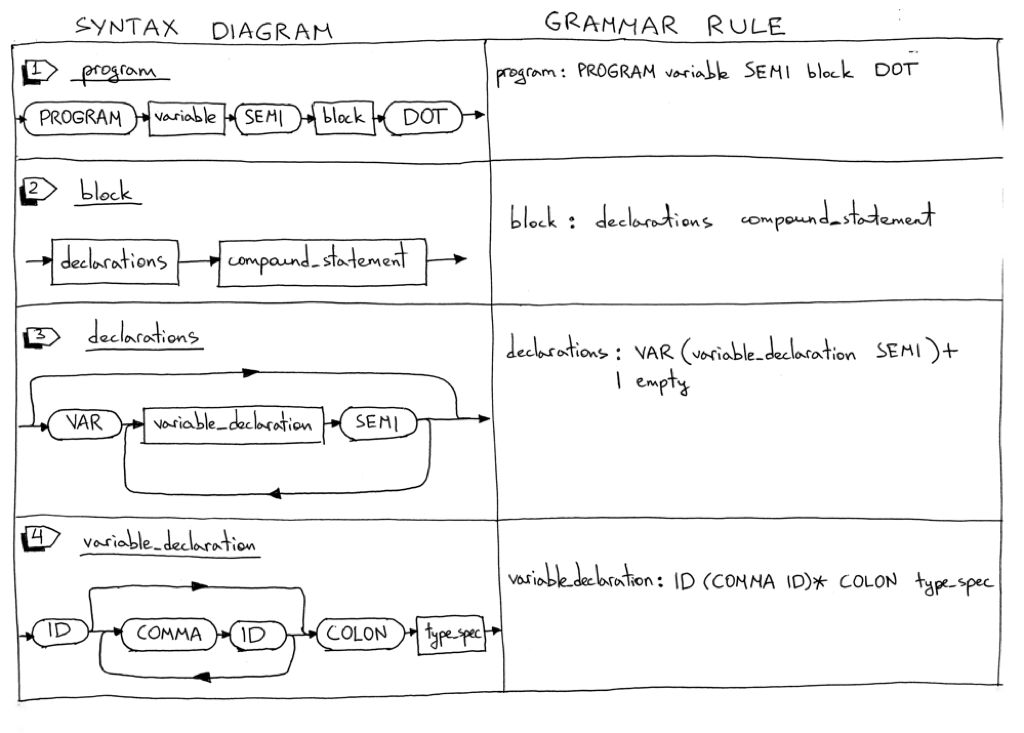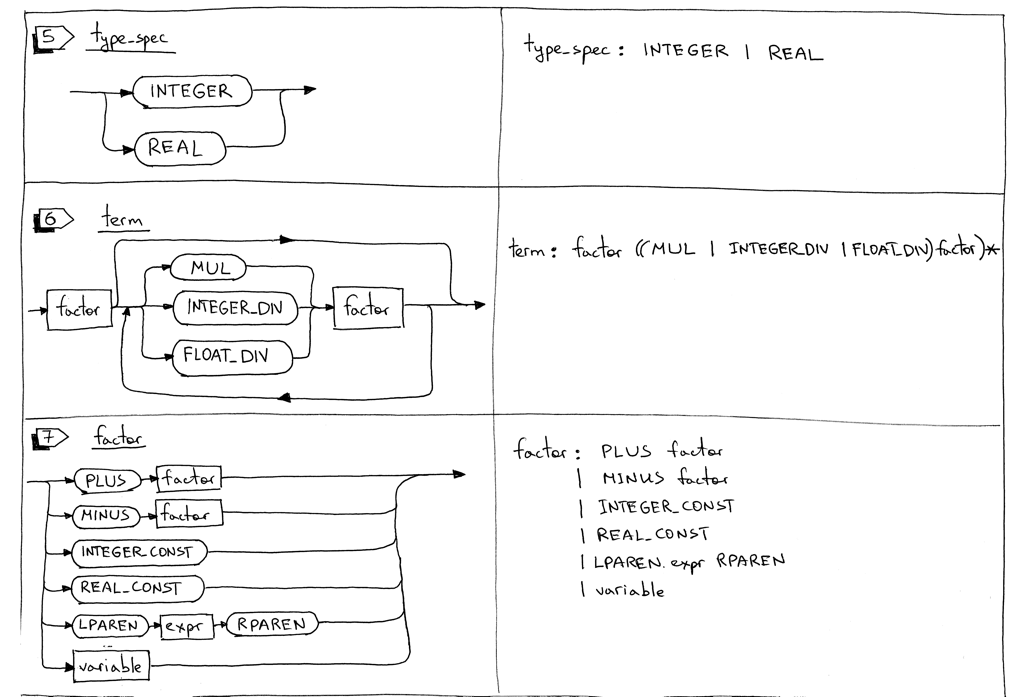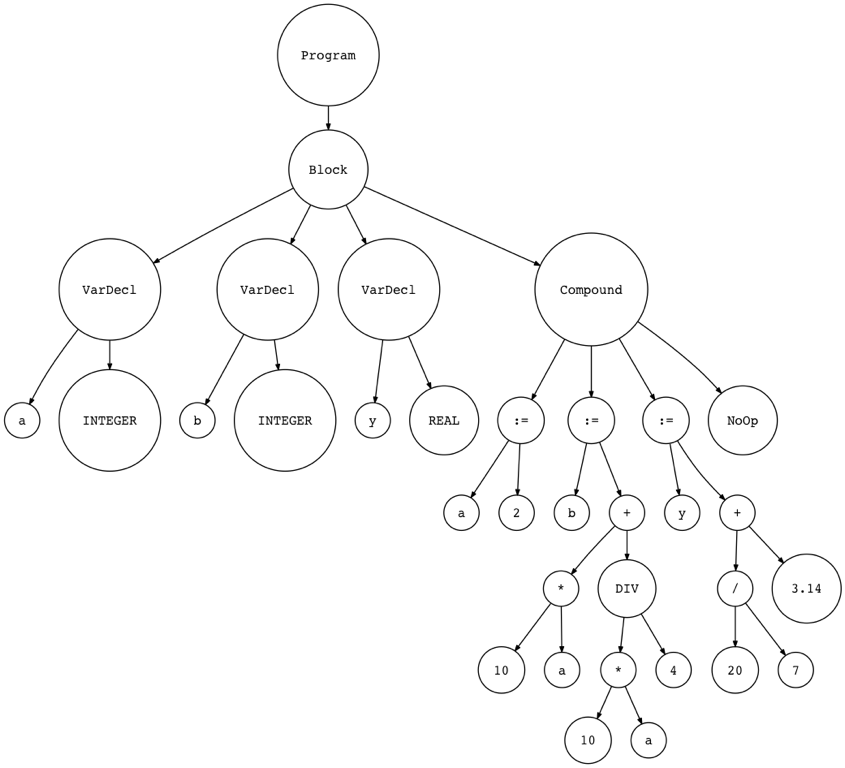Date

Today we will continue closing the gap between where we are right now and where we want to be: a fully functional interpreter for a subset of Pascal programming language.In this article we will update our interpreter to parse and interpret our very first complete Pascal program. The program can also be compiled by the Free Pascal compiler, fpc.

Here is the program itself:

```PROGRAM Part10;
VAR
number     : INTEGER;
a, b, c, x : INTEGER;
y          : REAL;

BEGIN {Part10}
BEGIN
number := 2;
a := number;
b := 10 * a + 10 * number DIV 4;
c := a - - b
END;
x := 11;
y := 20 / 7 + 3.14;
{ writeln('a = ', a); }
{ writeln('b = ', b); }
{ writeln('c = ', c); }
{ writeln('number = ', number); }
{ writeln('x = ', x); }
{ writeln('y = ', y); }
END.  {Part10}
```

Before we start digging into the details, download the source code of the interpreter from GitHub and the Pascal source code above, and try it on the command line:

```\$ python spi.py part10.pas
a = 2
b = 25
c = 27
number = 2
x = 11
y = 5.99714285714
```

If I remove the comments around the writeln statements in the part10.pas file, compile the source code with fpc and then run the produced executable, this is what I get on my laptop:

```\$ fpc part10.pas
\$ ./part10
a = 2
b = 25
c = 27
number = 2
x = 11
y =  5.99714285714286E+000
```

Okay, let’s see what we’re going cover today:

1. We will learn how to parse and interpret the Pascal PROGRAM header
2. We will learn how to parse Pascal variable declarations
3. We will update our interpreter to use the DIV keyword for integer division and a forward slash / for float division

Let’s dive in and look at the grammar changes first. Today we will add some new rules and update some of the existing rules.1. The program definition grammar rule is updated to include the PROGRAM reserved keyword, the program name, and a block that ends with a dot. Here is an example of a complete Pascal program:

```PROGRAM Part10;
BEGIN
END.
```
2. The block rule combines a declarations rule and a compound_statement rule. We’ll also use the rule later in the series when we add procedure declarations. Here is an example of a block:

```VAR
number : INTEGER;

BEGIN
END
```

Here is another example:

```BEGIN
END
```
3. Pascal declarations have several parts and each part is optional. In this article, we’ll cover the variable declaration part only. The declarations rule has either a variable declaration sub-rule or it’s empty.

4. Pascal is a statically typed language, which means that every variable needs a variable declaration that explicitly specifies its type. In Pascal, variables must be declared before they are used. This is achieved by declaring variables in the program variable declaration section using the VAR reserved keyword. You can define variables like this:

```VAR
number     : INTEGER;
a, b, c, x : INTEGER;
y          : REAL;
```
5. The type_spec rule is for handling INTEGER and REAL types and is used in variable declarations. In the example below

```VAR
a : INTEGER;
b : REAL;
```

the variable “a” is declared with the type INTEGER and the variable “b” is declared with the type REAL (float). In this article we won’t enforce type checking, but we will add type checking later in the series.

6. The term rule is updated to use the DIV keyword for integer division and a forward slash / for float division.

Before, dividing 20 by 7 using a forward slash would produce an INTEGER 2:

```20 / 7 = 2
```

Now, dividing 20 by 7 using a forward slash will produce a REAL (floating point number) 2.85714285714 :

```20 / 7 = 2.85714285714
```

From now on, to get an INTEGER instead of a REAL, you need to use the DIV keyword:

```20 DIV 7 = 2
```
7. The factor rule is updated to handle both integer and real (float) constants. I also removed the INTEGER sub-rule because the constants will be represented by INTEGER_CONST and REAL_CONST tokens and the INTEGER token will be used to represent the integer type. In the example below the lexer will generate an INTEGER_CONST token for 20 and 7 and a REAL_CONST token for 3.14 :

```y := 20 / 7 + 3.14;
```

Here is our complete grammar for today:

```    program : PROGRAM variable SEMI block DOT

block : declarations compound_statement

declarations : VAR (variable_declaration SEMI)+
| empty

variable_declaration : ID (COMMA ID)* COLON type_spec

type_spec : INTEGER | REAL

compound_statement : BEGIN statement_list END

statement_list : statement
| statement SEMI statement_list

statement : compound_statement
| assignment_statement
| empty

assignment_statement : variable ASSIGN expr

empty :

expr : term ((PLUS | MINUS) term)*

term : factor ((MUL | INTEGER_DIV | FLOAT_DIV) factor)*

factor : PLUS factor
| MINUS factor
| INTEGER_CONST
| REAL_CONST
| LPAREN expr RPAREN
| variable

variable: ID
```

In the rest of the article we’ll go through the same drill we went through last time:

1. Update the lexer
2. Update the parser
3. Update the interpreter

Updating the Lexer

Here is a summary of the lexer changes:

1. New tokens
2. New and updated reserved keywords
3. New skip_comment method to handle Pascal comments
4. Rename the integer method and make some changes to the method itself
5. Update the get_next_token method to return new tokens

Let’s dig into the changes mentioned above:

1. To handle a program header, variable declarations, integer and float constants as well as integer and float division, we need to add some new tokens - some of which are reserved keywords - and we also need to update the meaning of the INTEGER token to represent the integer type and not an integer constant. Here is a complete list of new and updated tokens:

• PROGRAM (reserved keyword)
• VAR (reserved keyword)
• COLON (:)
• COMMA (,)
• INTEGER (we change it to mean integer type and not integer constant like 3 or 5)
• REAL (for Pascal REAL type)
• INTEGER_CONST (for example, 3 or 5)
• REAL_CONST (for example, 3.14 and so on)
• INTEGER_DIV for integer division (the DIV reserved keyword)
• FLOAT_DIV for float division ( forward slash / )
2. Here is the complete mapping of reserved keywords to tokens:

```RESERVED_KEYWORDS = {
'PROGRAM': Token('PROGRAM', 'PROGRAM'),
'VAR': Token('VAR', 'VAR'),
'DIV': Token('INTEGER_DIV', 'DIV'),
'INTEGER': Token('INTEGER', 'INTEGER'),
'REAL': Token('REAL', 'REAL'),
'BEGIN': Token('BEGIN', 'BEGIN'),
'END': Token('END', 'END'),
}
```
3. We’re adding the skip_comment method to handle Pascal comments. The method is pretty basic and all it does is discard all the characters until the closing curly brace is found:

```def skip_comment(self):
while self.current_char != '}':
self.advance()  # the closing curly brace
```
4. We are renaming the integer method the number method. It can handle both integer constants and float constants like 3 and 3.14:

```def number(self):
"""Return a (multidigit) integer or float consumed from the input."""
result = ''
while self.current_char is not None and self.current_char.isdigit():
result += self.current_char

if self.current_char == '.':
result += self.current_char

while (
self.current_char is not None and
self.current_char.isdigit()
):
result += self.current_char

token = Token('REAL_CONST', float(result))
else:
token = Token('INTEGER_CONST', int(result))

```
5. We’re also updating the get_next_token method to return new tokens:

```def get_next_token(self):
while self.current_char is not None:
...
if self.current_char == '{':
self.skip_comment()
continue
...
if self.current_char.isdigit():
return self.number()

if self.current_char == ':':

if self.current_char == ',':
...
if self.current_char == '/':
...
```

Updating the Parser

Now onto the parser changes.

Here is a summary of the changes:

1. New AST nodes: Program, Block, VarDecl, Type
2. New methods corresponding to new grammar rules: block, declarations, variable_declaration, and type_spec.
3. Updates to the existing parser methods: program, term, and factor

Let’s go over the changes one by one:

1. We’ll start with new AST nodes first. There are four new nodes:

• The Program AST node represents a program and will be our root node

```class Program(AST):
def __init__(self, name, block):
self.name = name
self.block = block
```
• The Block AST node holds declarations and a compound statement:

```class Block(AST):
def __init__(self, declarations, compound_statement):
self.declarations = declarations
self.compound_statement = compound_statement
```
• The VarDecl AST node represents a variable declaration. It holds a variable node and a type node:

```class VarDecl(AST):
def __init__(self, var_node, type_node):
self.var_node = var_node
self.type_node = type_node
```
• The Type AST node represents a variable type (INTEGER or REAL):

```class Type(AST):
def __init__(self, token):
self.token = token
self.value = token.value
```
2. As you probably remember, each rule from the grammar has a corresponding method in our recursive-descent parser. Today we’re adding four new methods: block, declarations, variable_declaration, and type_spec. These methods are responsible for parsing new language constructs and constructing new AST nodes:

```def block(self):
"""block : declarations compound_statement"""
declaration_nodes = self.declarations()
compound_statement_node = self.compound_statement()
node = Block(declaration_nodes, compound_statement_node)
return node

def declarations(self):
"""declarations : VAR (variable_declaration SEMI)+
| empty
"""
declarations = []
if self.current_token.type == VAR:
self.eat(VAR)
while self.current_token.type == ID:
var_decl = self.variable_declaration()
declarations.extend(var_decl)
self.eat(SEMI)

return declarations

def variable_declaration(self):
"""variable_declaration : ID (COMMA ID)* COLON type_spec"""
var_nodes = [Var(self.current_token)]  # first ID
self.eat(ID)

while self.current_token.type == COMMA:
self.eat(COMMA)
var_nodes.append(Var(self.current_token))
self.eat(ID)

self.eat(COLON)

type_node = self.type_spec()
var_declarations = [
VarDecl(var_node, type_node)
for var_node in var_nodes
]
return var_declarations

def type_spec(self):
"""type_spec : INTEGER
| REAL
"""
token = self.current_token
if self.current_token.type == INTEGER:
self.eat(INTEGER)
else:
self.eat(REAL)
node = Type(token)
return node
```
3. We also need to update the program, term, and, factor methods to accommodate our grammar changes:

```def program(self):
"""program : PROGRAM variable SEMI block DOT"""
self.eat(PROGRAM)
var_node = self.variable()
prog_name = var_node.value
self.eat(SEMI)
block_node = self.block()
program_node = Program(prog_name, block_node)
self.eat(DOT)
return program_node

def term(self):
"""term : factor ((MUL | INTEGER_DIV | FLOAT_DIV) factor)*"""
node = self.factor()

while self.current_token.type in (MUL, INTEGER_DIV, FLOAT_DIV):
token = self.current_token
if token.type == MUL:
self.eat(MUL)
elif token.type == INTEGER_DIV:
self.eat(INTEGER_DIV)
elif token.type == FLOAT_DIV:
self.eat(FLOAT_DIV)

node = BinOp(left=node, op=token, right=self.factor())

return node

def factor(self):
"""factor : PLUS factor
| MINUS factor
| INTEGER_CONST
| REAL_CONST
| LPAREN expr RPAREN
| variable
"""
token = self.current_token
if token.type == PLUS:
self.eat(PLUS)
node = UnaryOp(token, self.factor())
return node
elif token.type == MINUS:
self.eat(MINUS)
node = UnaryOp(token, self.factor())
return node
elif token.type == INTEGER_CONST:
self.eat(INTEGER_CONST)
return Num(token)
elif token.type == REAL_CONST:
self.eat(REAL_CONST)
return Num(token)
elif token.type == LPAREN:
self.eat(LPAREN)
node = self.expr()
self.eat(RPAREN)
return node
else:
node = self.variable()
return node
```

Now, let’s see what the Abstract Syntax Tree looks like with the new nodes. Here is a small working Pascal program:

```PROGRAM Part10AST;
VAR
a, b : INTEGER;
y    : REAL;

BEGIN {Part10AST}
a := 2;
b := 10 * a + 10 * a DIV 4;
y := 20 / 7 + 3.14;
END.  {Part10AST}
```

Let’s generate an AST and visualize it with the genastdot.py:

```\$ python genastdot.py part10ast.pas > ast.dot && dot -Tpng -o ast.png ast.dot
```In the picture you can see the new nodes that we have added.

Updating the Interpreter

We’re done with the lexer and parser changes. What’s left is to add new visitor methods to our Interpreter class. There will be four new methods to visit our new nodes:

• visit_Program
• visit_Block
• visit_VarDecl
• visit_Type

They are pretty straightforward. You can also see that the Interpreter does nothing with VarDecl and Type nodes:

```def visit_Program(self, node):
self.visit(node.block)

def visit_Block(self, node):
for declaration in node.declarations:
self.visit(declaration)
self.visit(node.compound_statement)

def visit_VarDecl(self, node):
# Do nothing
pass

def visit_Type(self, node):
# Do nothing
pass
```

We also need to update the visit_BinOp method to properly interpret integer and float divisions:

```def visit_BinOp(self, node):
if node.op.type == PLUS:
return self.visit(node.left) + self.visit(node.right)
elif node.op.type == MINUS:
return self.visit(node.left) - self.visit(node.right)
elif node.op.type == MUL:
return self.visit(node.left) * self.visit(node.right)
elif node.op.type == INTEGER_DIV:
return self.visit(node.left) // self.visit(node.right)
elif node.op.type == FLOAT_DIV:
return float(self.visit(node.left)) / float(self.visit(node.right))
```

Let’s sum up what we had to do to extend the Pascal interpreter in this article:

• Add new rules to the grammar and update some existing rules
• Add new tokens and supporting methods to the lexer, update and modify some existing methods
• Add new AST nodes to the parser for new language constructs
• Add new methods corresponding to the new grammar rules to our recursive-descent parser and update some existing methods
• Add new visitor methods to the interpreter and update one existing visitor method

As a result of our changes we also got rid of some of the hacks I introduced in Part 9, namely:

• Our interpreter can now handle the PROGRAM header
• Variables can now be declared using the VAR keyword
• The DIV keyword is used for integer division and a forward slash / is used for float division

If you haven’t done so yet, then, as an exercise, re-implement the interpreter in this article without looking at the source code and use part10.pas as your test input file.

That’s all for today. In the next article, I’ll talk in greater detail about symbol table management. Stay tuned and see you soon!